3.4 Pythagorean Scales

Intervals to scales

In Section 3.2, we introduced the concept of intervals and how one goes up and down by a certain interval. However, Pythagoras’s real goal was to explain the musical scale, not just intervals. To this end, he came up with a very simple process for generating the scale based on intervals, in fact, using just two intervals, the octave and the Perfect Fifth.

The method is as follows: we start on any note, in this example we will use D. This is the first note of the scale. If we go up by an octave, we again reach a D, one octave higher. This defines the range of the scale that we want to create. We want to fill in the notes of the scale between these two D’s.

To get the next note, we start on the original D and go up by a Perfect Fifth. This gives us an A. We can also go down by a Perfect Fifth that takes us to a G below the original D. Although this is a new note, it is not in the range that we specified above. So, we simply raise it by an octave, which does not change the musical characteristic of the note. So far, we have D, G, A, D. Notice that these are just the notes we discussed in Section 3.2, but now we can keep going.

From A, we can again go up by a Perfect Fifth, which takes us to E. However, this note is again outside the original scale that we are creating, so we bring the note down by an octave. Similarly, from G we go down by a Perfect Fifth to C and bring it up by an octave. The notes that we now have are D, E, G, A, C, D. If we play these notes on a keyboard, you may recognize them as a pentatonic scale. This scale is used by many cultures, especially Asian ones.

Perhaps you can now see the process. Going up and down by Perfect Fifths generates new notes. However, sometimes these notes are outside the original scale that we are trying to create. Whenever a new note is outside this range we move it up or down by an octave to put it in range.

You may not be familiar with the names of the notes, and this is not so important. What is very important is to know the frequency of each note with respect to the original note of the scale. So, we will review the process but now keeping track of the frequencies of each note. Since the ratios start to get complicated we will also convert them to simple decimal notation.

This is the range of the scale that we are trying to create. Since we only care about the relationships between the notes, we give the first note a frequency of 1: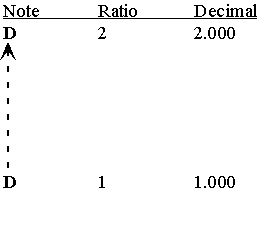Now lets add notes by going up and down by a Perfect Fifth from the D: solid arrows are Perfect Fifths, dashed arrows are octaves.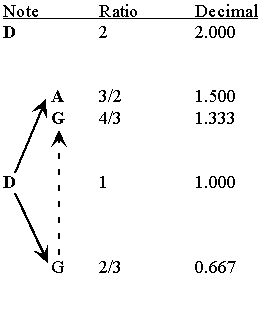This gives the notes D, G, A, D, and the ratios 1, 4/3, 3/2, 2. If we continue: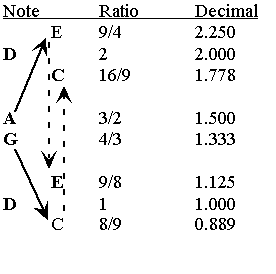This is the pentatonic scale, D, E, G, A, C, D. If we do this once more, we get the Western scale, also called the diatonic scale.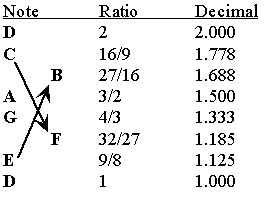This is the Western scale starting on D. However, we could have started on any note and create the scale in the same way.

Circle of Fifths

What happens if we keep on going? This way of adding notes by going up and down by Perfect Fifths can be organized in a diagram called the Circle of Fifths: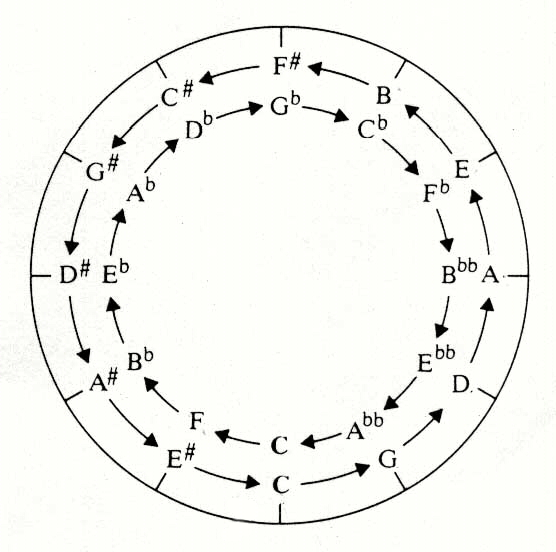It shows what note you arrive at by going up or down a fifth from any other note. As you can see, the outer circle has more than the seven notes that we have already generated. This means that we stopped too soon. If we repeat the process a few more times, we arrive at the chromatic scale. This is the scale you hear if you play every note on the piano, white notes and black notes. There are 12 notes in the chromatic scale.

And if we keeping adding more notes in the same way? Do we start repeating? The answer is Yes and No. The question is whether the inner circle in the Circle of Fifths is the same as the outer circle. In other words, is a D-sharp the same as an E-flat? On a piano, they are the same, but the exact frequency that you arrive at using the Pythagorean system gives different values for these two notes. So, they are not exactly the same. If fact, the Arabic scale considers these notes to be different. This is not a scale that we are familiar with in the West. It contains notes in between our smallest division and has many more notes than we are used to, about 24 notes in the scale. However, it is perfectly normal in Arabic music.

Does this process ever stop? Can we keep adding notes indefinitely? The answer is Yes, we can always keep generating new notes in this way! For the process to stop, it would have to start repeating at some point. In other words, if we eventually got back to D, we would then simply start over again and not get any new notes. However, this will never happen. The reasoning is a little tricky: say we always go up by Perfect Fifths. If we go up n times, the frequency is (3/2)n. Now we go down by octaves m times. This takes us to (1/2)m. Our final frequency is (3/2)n(1/2)m. Can this ratio ever equal 1. Can we ever get back to the starting note? For (3/2)n(1/2)m = 1, it would have to be true that (3/2)n = 2m. However, 2m is always an integer, while (3/2)n is never an integer. Therefore, it is never true that (3/2)n = 2m. Thus, we always keep adding new notes. Eventually, the notes get so close together that we will note be able to hear the difference, but we will return to this question later in the course.

Returning to the Western scale, there is another important feature of a scale, and that is the interval between adjacent notes in the scale. Here, again, is our final Western scale, including the interval between each note:

 Note Decimal Ratio Interval between notes D 2.000 2 9/8 C 1.778 16/9 256/243 B 1.688 27/16 9/8 A 1.500 3/2 9/8 G 1.333 4/3 9/8 F 1.185 32/27 256/243 E 1.125 9/8 9/8 D 1.000 1

The ratios of the individual notes are getting complicated and the intervals between the notes are getting quite complicated, but, amazingly, there are only two such intervals, 9/8 and 256/243! We can add these new intervals to our list. They are called the whole step and the half step, respectively:

Whole step = 9/8

Half step = 256/243

This is a good check that the system is working. The idea of a scale is that there is some regular pattern to the sequence of notes in a scale. You may have noticed that the intervals between the notes of a scale are not all equal, that would lead to a rather uninteresting scale. But, they are not all different, either, as that would be very confusing. As it turns out, the Pythagorean scale is made up of whole steps and half steps in a particular pattern. There is one small difference from what we might have expected. From their names, you might think that two half steps is equal to one whole step. Is this true?